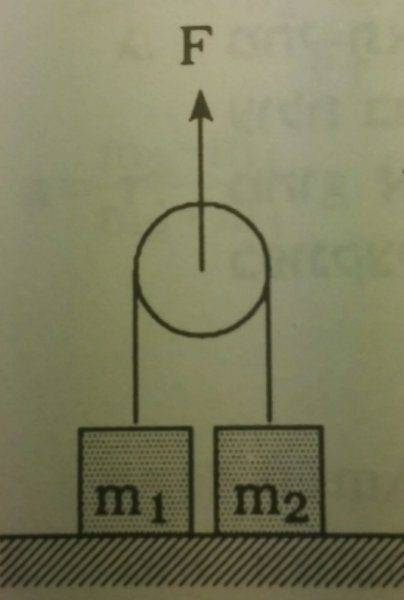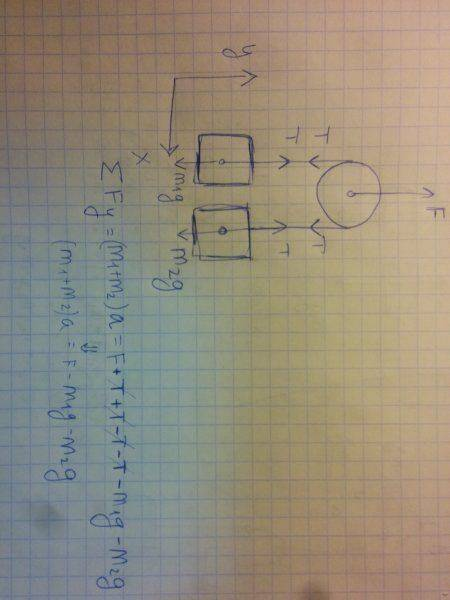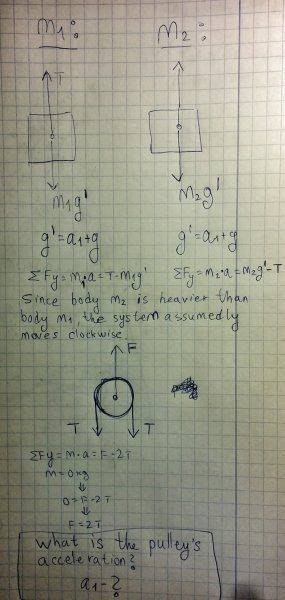# Pulley suspending two different masses lifted upwardsThe force F pulls upwards two masses, m1 and m2(m2>m1), which are connected to each othet via a massless pulley. The masses are perched on the ground before movement starts.

A. What is the acceleration of each one of the respective masses in each of the following situations:
1)F<2*m1*g
2)2*m1*g<F<2*m2*g
3)2*m2*g<F

B. What is the rope's tension in each situation?

Though I'd viewed similar questions posted here, I couldn't draw any solution to this particular case involving as many parmeters as it does. Also, since, as I understand it, the heavier mass doesn't accelerate at all during the first stages of movement, but later does, I didn't grasp how to calculate the acceleration.
I assumed that I should first treat both masses as one, and then address each seperately - though I wasn't sure how to proceed in that direction.(sorry for the inverted image)
Any help would be appreciated.

Thank you.

BvU
Homework Helper
Hello Sabatie, welcome to PF :)

Did you notice the template ? Pity it's gone now. It helps you to provide some essential information to potential helpers. I, for example, now have no idea whatsoever what you mean by a. The template provides space for all variables and given/known data. And I also have no idea if you know which equations you need to work out this exercise. The so-called Relevant equations.

1. The problem statement, all variables and given/known data
2. Relevant equations

3. The Attempt at a Solution

Yes, there is something, but it now hangs loose in the air.
Which, as it happens, is a hint for you: make free-body diagrams for the three parts separately and then connect them.

On the bright side:
You are well under way. It's just that you haven't pinned down a. What is it and what is the relationship between your a and what is really asked for in part A of the exercise ? (Read it again, word for word...)

Hello Sabatie, welcome to PF :)

Did you notice the template ? Pity it's gone now. It helps you to provide some essential information to potential helpers. I, for example, now have no idea whatsoever what you mean by a. The template provides space for all variables and given/known data. And I also have no idea if you know which equations you need to work out this exercise. The so-called Relevant equations.

1. The problem statement, all variables and given/known data
2. Relevant equations

3. The Attempt at a Solution

Yes, there is something, but it now hangs loose in the air.
Which, as it happens, is a hint for you: make free-body diagrams for the three parts separately and then connect them.

On the bright side:
You are well under way. It's just that you haven't pinned down a. What is it and what is the relationship between your a and what is really asked for in part A of the exercise ? (Read it again, word for word...)

Hello, and thank you for the welcome and reply. I apologize for not adhering to the template; I sincerely thought I had framed my question comprehensively.

Here are the diagrams:I would appreciate it if you could shed some light on how I am to find the pulley's acceleration and, by doing so - find each body's acceleration(which are identical, since they move correspondingly).

haruspex